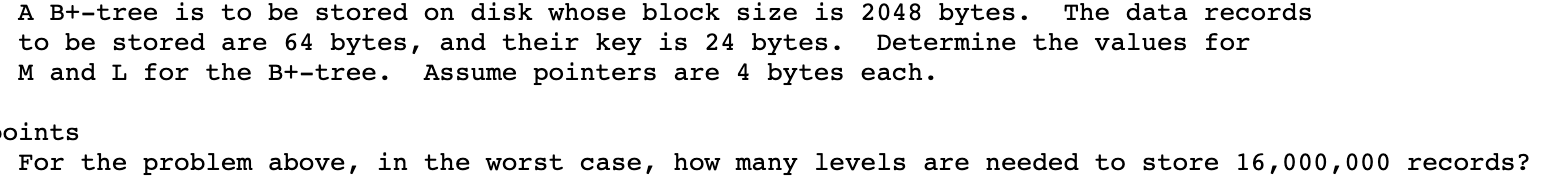# A B+-tree is to be stored on disk whose block size is 2048 bytes.to be stored are 64 bytes, and their key is 24 bytes.The data recordsDetermine the values forM and L for the B+-tree.Assume pointers are 4 bytes each.ointsFor the problem above, in the worst case, how many levels are needed to store 16,000,000 records?

Question
1 viewshelp_outlineImage TranscriptioncloseA B+-tree is to be stored on disk whose block size is 2048 bytes. to be stored are 64 bytes, and their key is 24 bytes. The data records Determine the values for M and L for the B+-tree. Assume pointers are 4 bytes each. oints For the problem above, in the worst case, how many levels are needed to store 16,000,000 records? fullscreen
check_circle

Warm-Up Description –

B Tree – In this binary search tree, a node can have more than 2 children and is a self-balancing tree. In this, the data is sorted and the operations like insertion, searching, and deletion can be performed.

Key – in the general term, the key is a collection of values. In B tree, the key is a value that divides the B tree into the sub-trees.

M – This is an order of a tree where the non-leaf will have M number of children.

L - L is the number of records to be stored in each leaf.

Values for M and L –

Data are given –

Block size = 2048 bytes

Data records to be stored = 64 bytes

Key  = 24 bytes

Pointer = 4 bytes

For L,

Using the formula –

L = block size / data records

Putting the values

L = 2048 / 64

L = 32

Therefore, 32 records can be stored per leaf.

For M,

Using the formula –

Pointer * M + key * (...

### Want to see the full answer?

See Solution

#### Want to see this answer and more?

Solutions are written by subject experts who are available 24/7. Questions are typically answered within 1 hour.*

See Solution
*Response times may vary by subject and question.
Tagged in